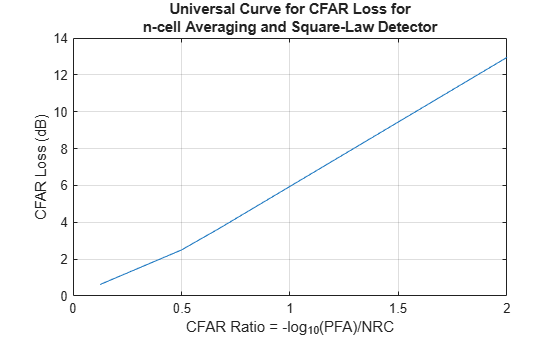cfarloss

Loss due to constant false alarm rate (CFAR) adaptive processing

Description

example

LCFAR = cfarloss(PFA,NRC) computes approximated CFAR loss, LCFAR, in dB for the probability of false alarm, PFA, and number of reference cells, NRC, that you specify. The function calculates loss for the cell-averaging (CA) CFAR method and a square-law detector based on the Gregers-Hansen's universal CFAR loss curve.

LCFAR = cfarloss(PFA,NRC,Name,Value) specifies additional options using name-value arguments. For example, LCFAR = cfarloss(1e-8,4:4:64,'Method','CA') computes approximate loss using the CA CFAR process.. You can specify multiple name-value arguments.

Examples

collapse all

Calculate the CFAR loss for an n-cell averaging and a square-law detector. Assume the numbers of reference cells from 4–64 and the probability of false alarm of 1e-8.

PFA = 1e-8;
NRC = 4:4:64;
LCFAR = cfarloss(PFA,NRC);

Plot the resulting loss vs CFAR ratio. The CFAR ratio is calculated using the equation, X = -${\mathrm{log}}_{10}$(PFA)/NRC.

plot(-log10(PFA)./NRC,LCFAR)
grid on;
xlabel('CFAR Ratio = -log_{10}(PFA)/NRC');
ylabel('CFAR Loss (dB)');
title({'Universal Curve for CFAR Loss for',...
'n-cell Averaging and Square-Law Detector'});Input Arguments

collapse all

Probability of false alarm, specified as a positive scalar in the range [1e-15,1e-3] or as a length-K vector with each element in the range [1e-15,1e-3] .

Number of reference cells used in CFAR processing, specified as a positive scalar or length-K vector.

Name-Value Arguments

Specify optional comma-separated pairs of Name,Value arguments. Name is the argument name and Value is the corresponding value. Name must appear inside quotes. You can specify several name and value pair arguments in any order as Name1,Value1,...,NameN,ValueN.

Example: LCFAR = cfarloss(1e-8,4:4:64,'DetectorType','Log')

Type of CFAR process, specified as a either 'CA' for cell-averaging process or 'GOCA' for greatest-of cell-averaging process.

Example: 'Method','GOCA'

Type of detector in use, specified as either 'SquareLaw', 'Linear', or 'Log'.

Example: 'DetectorType','Linear'

Output Arguments

collapse all

CFAR loss, returned as a K-element vector in dB.

Extended Capabilities

C/C++ Code GenerationGenerate C and C++ code using MATLAB® Coder™.